# NCERT Solutions, Determinants, Part -7, Class 12, Maths Notes - Class 12

## Class 12: NCERT Solutions, Determinants, Part -7, Class 12, Maths Notes - Class 12

The document NCERT Solutions, Determinants, Part -7, Class 12, Maths Notes - Class 12 is a part of Class 12 category.
All you need of Class 12 at this link: Class 12

Determinants

Miscellaneous Solutions

Question 1:
Prove that the determinant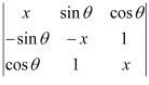is independent of θ.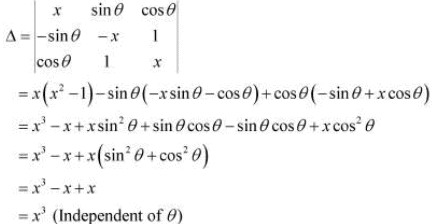Hence, Δ is independent of θ.

Question 2: Without expanding the determinant, prove that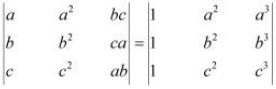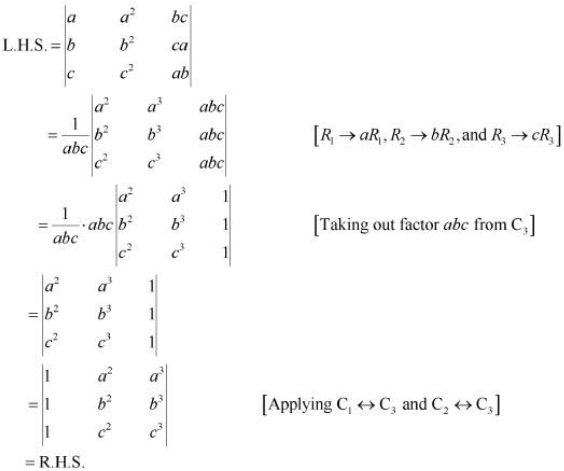Hence, the given result is proved.

Question 3: Evaluate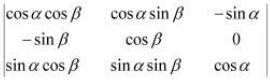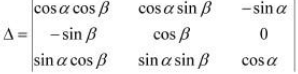Expanding along C3, we have: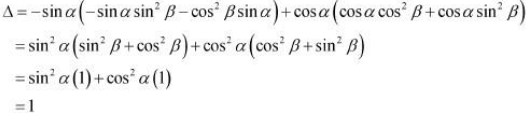Question 4: If a, b and c are real numbers, and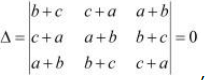,
Show that either a + b + c = 0 or a = b = c.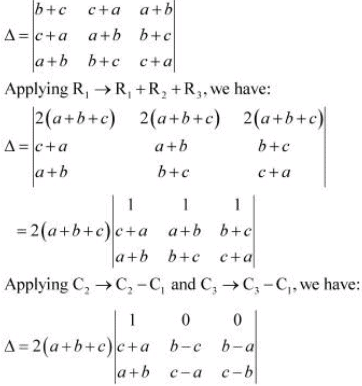Expanding along R1, we have: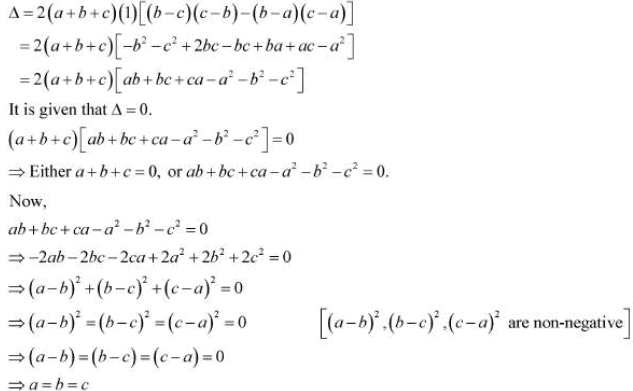Hence, if Δ = 0, then either a + b + c = 0 or a = b = c.

Question 5: Solve the equations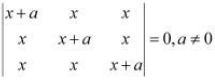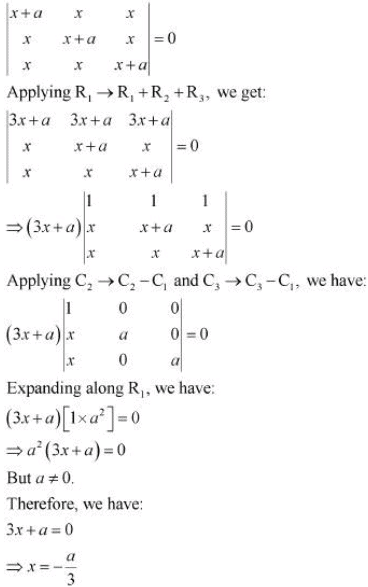Question 6: Prove that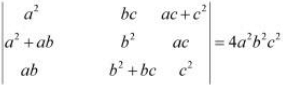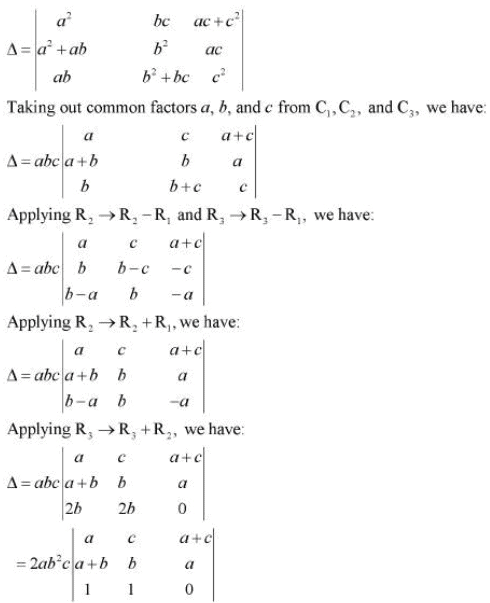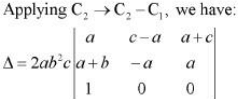Expanding along R3, we have: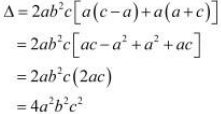Hence, the given result is proved.

Question 8: Let verify that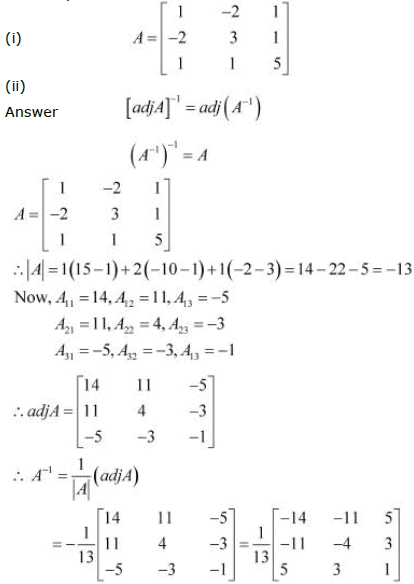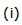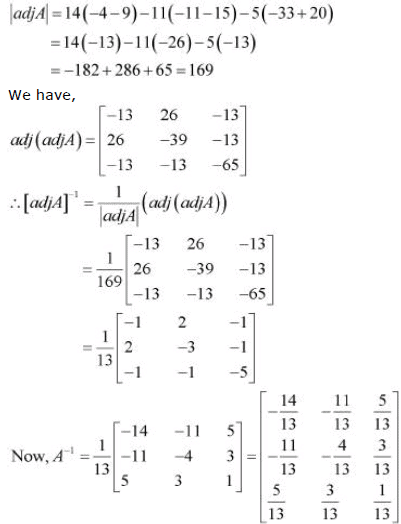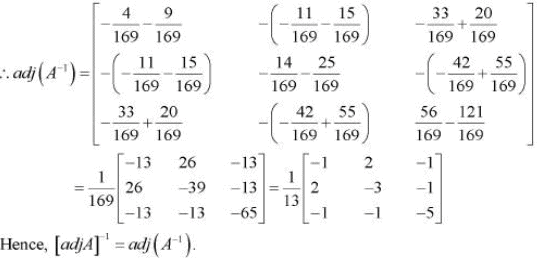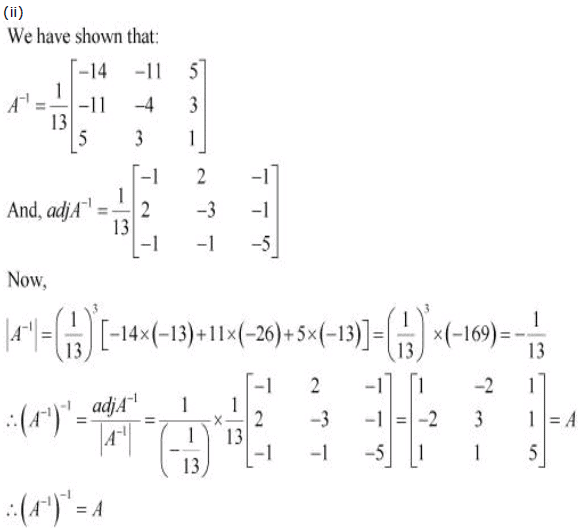Question 9: Evaluate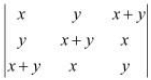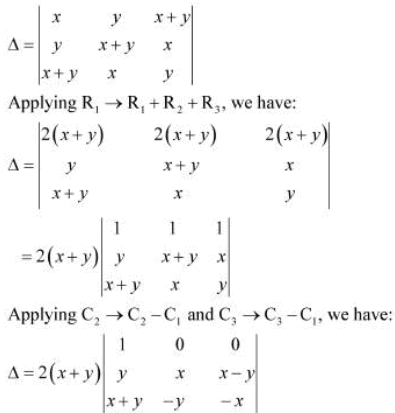Expanding along R1, we have: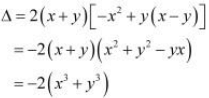Question 10: Evaluate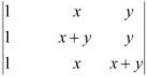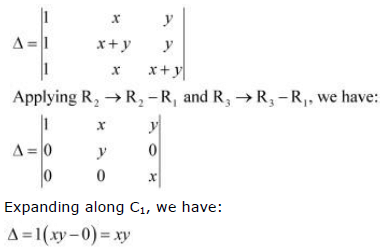Question 11: Using properties of determinants, prove that: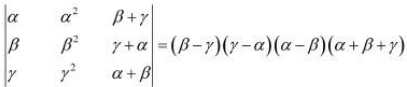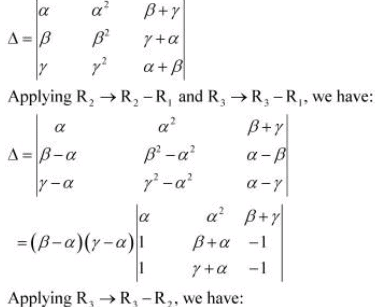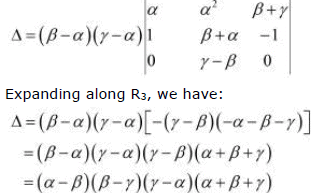Hence, the given result is proved.

Question 12: Using properties of determinants, prove that: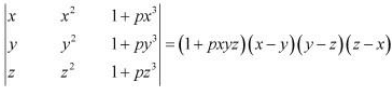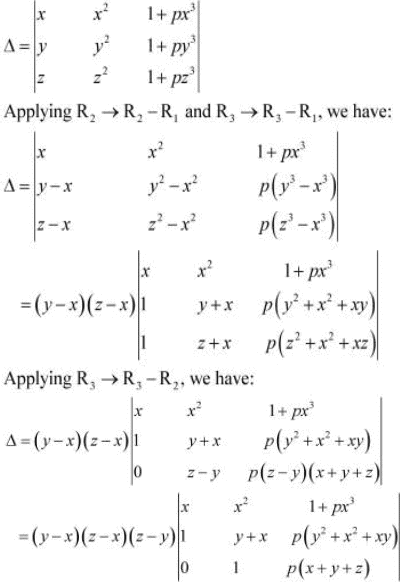Expanding along R3, we have: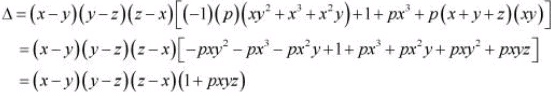Hence, the given result is proved.

Question 13: Using properties of determinants, prove that: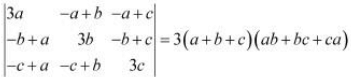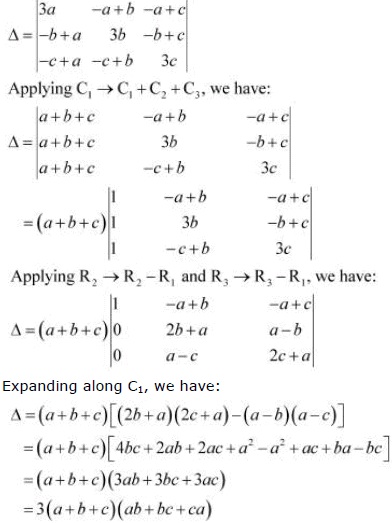Hence, the given result is proved.

Question 14: Using properties of determinants, prove that: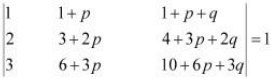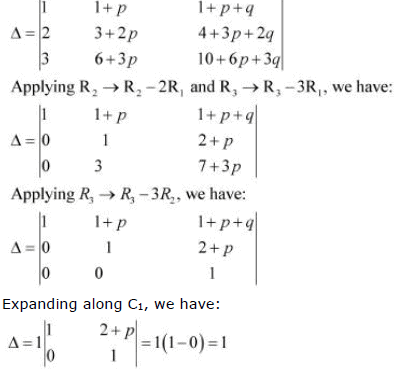Hence, the given result is proved.

Question 15: Using properties of determinants, prove that: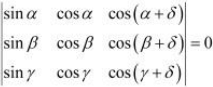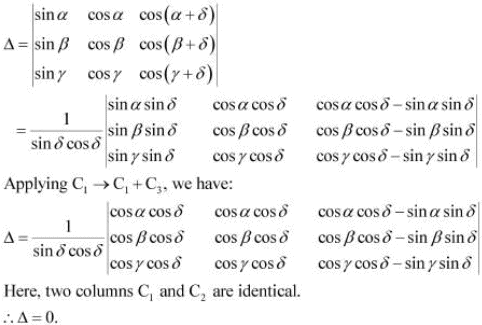Hence, the given result is proved.

Question 16: Solve the system of the following equations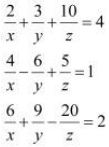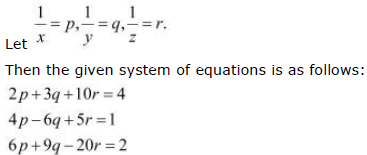This system can be written in the form of AX = B, where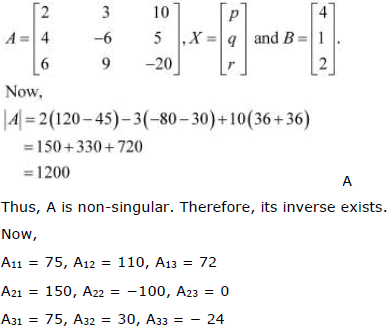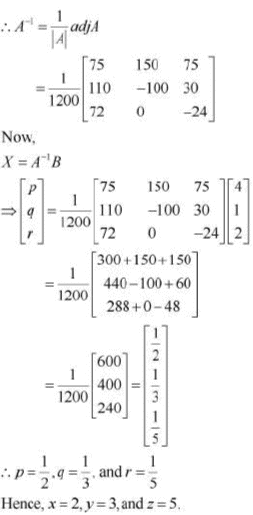Question 17: Choose the correct answer. If a, b, c, are in A.P., then the determinant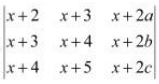A. 0

B. 1

C. x

D. 2x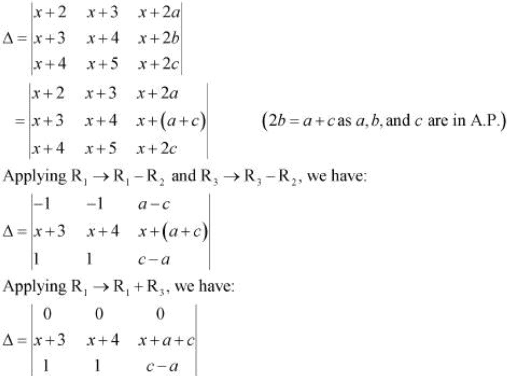Here, all the elements of the first row (R1) are zero.
Hence, we have Δ = 0.

Question 18: Choose the correct answer. If x, y, z are nonzero real numbers, then the inverse of matrix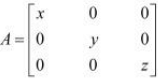is
A.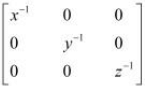B.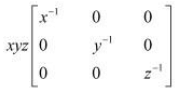C.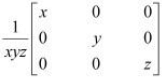D.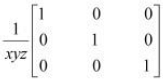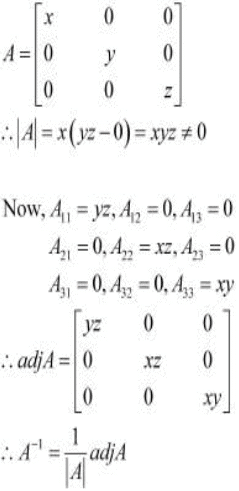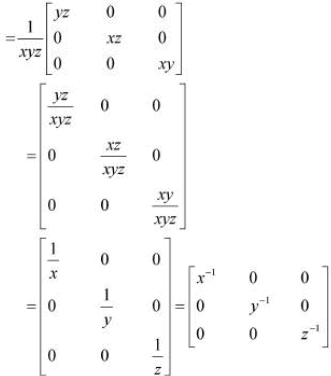Question 19: Choose the correct answer.
Let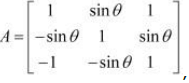, where 0 ≤ θ≤ 2π, then
A). Det (A) = 0
B). Det (A) (2, ∞)
C). Det (A) (2, 4)
D). Det (A) [2, 4]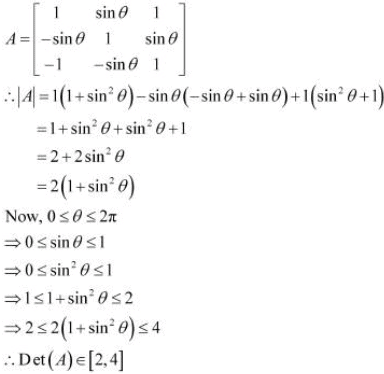The document NCERT Solutions, Determinants, Part -7, Class 12, Maths Notes - Class 12 is a part of Class 12 category.
All you need of Class 12 at this link: Class 12Use Code STAYHOME200 and get INR 200 additional OFF

Track your progress, build streaks, highlight & save important lessons and more!

,

,

,

,

,

,

,

,

,

,

,

,

,

,

,

,

,

,

,

,

,

,

,

,

,

,

,

,

,

,

,

,

,

;